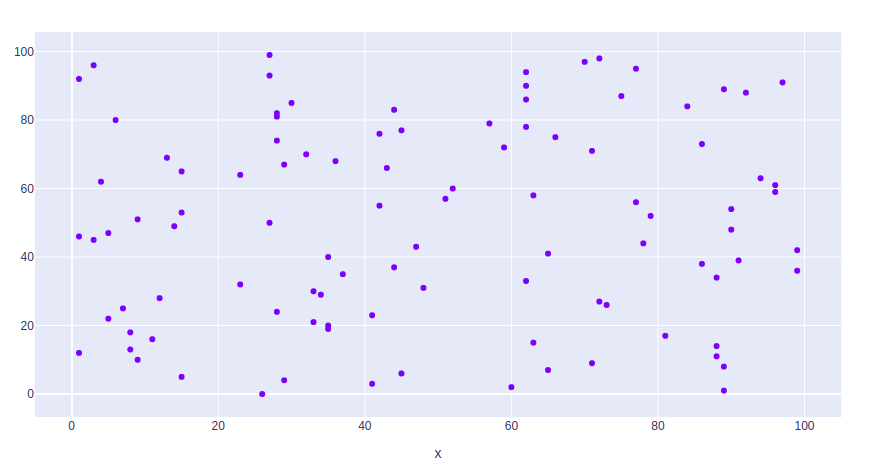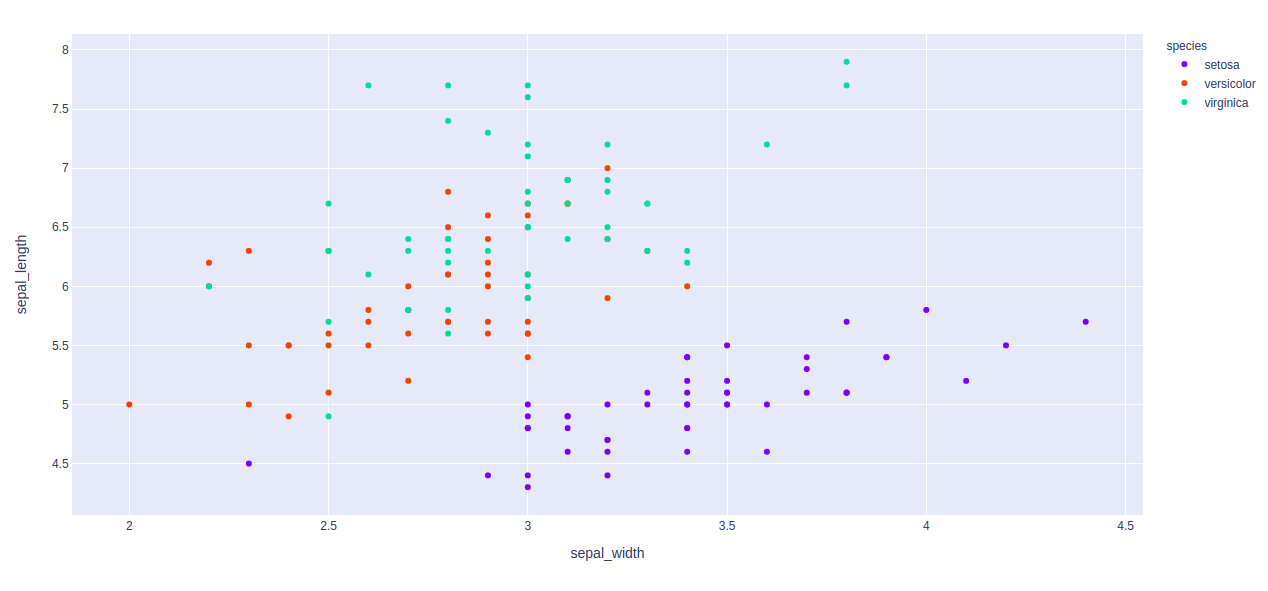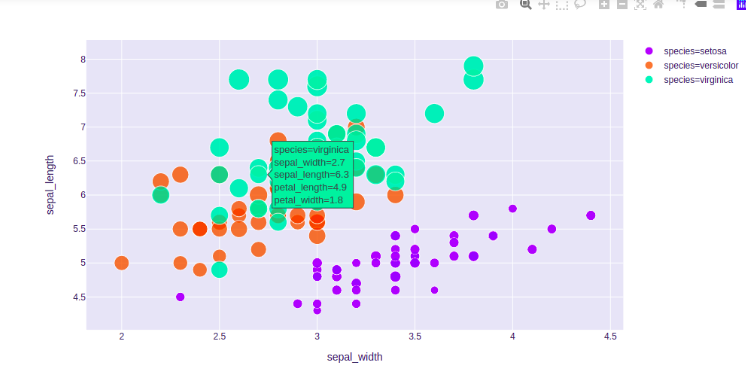Related Articles

# Scatter plot using Plotly in Python

• Last Updated : 10 Jul, 2020

Plotly is a Python library which is used to design graphs, especially interactive graphs. It can plot various graphs and charts like histogram, barplot, boxplot, spreadplot and many more. It is mainly used in data analysis as well as financial analysis. Plotly is an interactive visualization library.

## Scatter Plot

A scatter plot is a diagram where each value is represented by the dot graph. Scatter  plot needs arrays for the same length, one for the value of x-axis and other value for the y-axis. Each data is represented as a dot point, whose location is given by x and y columns. It can be created using the scatter() method of plotly.express

Syntax: plotly.express.scatter(data_frame=None, x=None, y=None, color=None, symbol=None, size=None, hover_name=None, hover_data=None, custom_data=None, text=None, facet_row=None, facet_col=None, facet_col_wrap=0, error_x=None, error_x_minus=None, error_y=None, error_y_minus=None, animation_frame=None, animation_group=None, category_orders={}, labels={}, orientation=None, color_discrete_sequence=None, color_discrete_map={}, color_continuous_scale=None, range_color=None, color_continuous_midpoint=None, symbol_sequence=None, symbol_map={}, opacity=None, size_max=None, marginal_x=None, marginal_y=None, trendline=None, trendline_color_override=None, log_x=False, log_y=False, range_x=None, range_y=None, render_mode=’auto’, title=None, template=None, width=None, height=None)

Parameters:

Example:

## Python3

 `import` `plotly.express as px``import` `numpy as np ``   ` ` ` `# creating random data through randomint ``# function of numpy.random ``np.random.seed(``42``) ``   ` `random_x``=` `np.random.randint(``1``,``101``,``100``) ``random_y``=` `np.random.randint(``1``,``101``,``100``) ``   ` `plot ``=` `px.scatter(random_x, random_y)``plot.show()`

Output:## Changing color

Color of dots of the scatter plot can be changed using the color argument of the scatter() method.

Example: We will use the built-in iris dataset.

## Python3

 `import` `plotly.express as px`` ` `# Loading the iris dataset``df ``=` `px.data.iris()`` ` `fig ``=` `px.scatter(df, x``=``"sepal_width"``, y``=``"sepal_length"``,``                 ``color``=``"species"``)``fig.show()`

Output:## Changing Size

The size of the dots of the scatter plot can be changed using the size argument of the scatter() method.

Example:

## Python3

 `import` `plotly.express as px`` ` `# Loading the iris dataset``df ``=` `px.data.iris()`` ` `fig ``=` `px.scatter(df, x``=``"sepal_width"``, y``=``"sepal_length"``,``                 ``color``=``"species"``, size``=``'petal_length'``, ``                 ``hover_data``=``[``'petal_width'``])``fig.show()`

Output:Attention geek! Strengthen your foundations with the Python Programming Foundation Course and learn the basics.

To begin with, your interview preparations Enhance your Data Structures concepts with the Python DS Course. And to begin with your Machine Learning Journey, join the Machine Learning – Basic Level Course

My Personal Notes arrow_drop_up